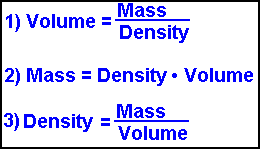Density Mass & Volume
Ultra Calculator
***** Weight of Water Calculator *****
Scroll to the bottom for instructions

Do you want to solve for:
Volume     Mass     or Density ?
Weight of Water

 VOLUME *** V O L U M E ************** Cubic Millimeters Milliliters = Cubic Centimeters Cubic Inches Fluid Ounces Liters Gallons (US) Cubic Feet Cubic Yards Cubic Meters
 MASS *** M A S S ********************* Milligrams Grains Grams Ounces Pounds Kilograms Tons-Short (2,000 Pounds) Tons-Metric (1,000 Kilograms) Tons-Long (2,240 Pounds)
 DENSITY *** D E N S I T Y *********************** Grains / Gallon Grams/Liter = kg/m^3 (stere) Pounds/Cubic Foot Pounds/Gallon Ounces (Mass) / Ounces(Volume) H2O DENSITY = gm/cc = gm/ml = kg/l = tonne/stere Short Tons (2,000 Lbs) / Cubic Yard Long Tons (2,240 Lbs) / Cubic Yard Ounces (Mass) / Cubic Inch

I N S T R U C T I O N S
Density of Water   = 1 g / cm3   =   1 kg / liter   =   1,000 kg / m3
Density of Air at 15°C   = .001225 g / cm3  =   .001225 kg / liter   =   1.225 kg / m3Density is generally stated as grams per cubic centimeter or kilograms per liter.
When using the International System of Units (SI), density is stated as kilograms per cubic meter.
The above 3 formulas are used for solving problems involving volume, mass and density. If you know 2 of the 3 variables the third can be calculated.
As usual, here at www.1728.com, we have a calculator that will do all the work for you.

This ultra calculator is special by allowing you to choose among a great variety of units (9 per category). Unlike other calculators, you are NOT confined to inputting volume in liters, mass in kilograms, etc. making this calculator quite versatile. Let's see some examples.

 1) How much volume does a ton of water occupy? FIRST CLICK ON WHAT YOU ARE SOLVING FOR - VOLUME Enter 1 in the mass box and choose Short Tons from its menu. Enter 1 in the density box and choose H2O Density from its menu. Click CALCULATE and your answer is 32.036 cubic feet.
2) The density (specific gravity) of gold is 19.3. How much is the weight of one cubic foot?
The most important step in using this calculator is:
FIRST CHOOSE WHAT YOU ARE SOLVING FOR.
In this case we are solving for weight or MASS so click on that button.
Enter 1 in the "volume" box and choose cubic feet from its menu.
Enter 19.3 in the "density" box and choose H2O Density from its menu.
Click the CALCULATE button and you will see this weighs 1,204.85 pounds. PLUS you get to see the answer in 8 other different units !!

3) You are measuring 15 cubic centimeters of a liquid that has a mass of 17 grams. What is its density?

FIRST CLICK ON WHAT YOU ARE SOLVING FOR - DENSITY
Enter 15 in the volume box and choose cubic centimeters from its menu.
Enter 17 in the mass box and choose grams from its menu.
Click CALCULATE and your answer is 1.133 that of H2O Density (as well as 8 other units).

***** Weight of Water Calculator *****
4) What is the weight of 3.5 gallons of water?

FIRST CLICK ON WHAT YOU ARE SOLVING FOR - Weight of Water
The density input box automatically gets filled in with the value of 1 and so you only have to enter one number.
Enter 3.5 in the volume input box then select "Gallons" from its menu.
Click "CALCULATE" and you will see that 3.5 gallons of water weighs 29.029 pounds (as well as 8 other units).

Here's hoping this calculator helps you with those math problems.

Significant Figures >>>
For easy readability, the answers are displayed in a "significant figure" format so you will not see answers such as 77.3333333333333333.
Numbers larger than 1,000 will be displayed in scientific notation and with the same number of significant figures specified. You may change the significant figures displayed by changing the number in the box above.
Internet Explorer and most other browsers, will display the answers properly but there are a few browsers that will show no output whatsoever. If so, enter a zero in the box above. This eliminates all formatting but it is better than seeing no output at all.